# infer

Infer vector error-correction (VEC) model innovations

## Syntax

``E = infer(Mdl,Y)``
``Tbl2 = infer(Mdl,Tbl1)``
``___ = infer(___,Name,Value)``
``````[___,logL] = infer(___)``````

## Description

example

````E = infer(Mdl,Y)` returns a numeric array `E` containing the series of multivariate inferred innovations from evaluating the fully specified VEC(p – 1) model `Mdl` at the numeric array of response data `Y`. For example, if `Mdl` is a VEC model fit to the response data `Y`, `E` contains the residuals.```

example

````Tbl2 = infer(Mdl,Tbl1)` returns the table or timetable `Tbl2` containing the multivariate residuals from evaluating the fully specified VEC(p – 1) model `Mdl` at the response variables in the table or timetable of data `Tbl1`. (since R2022b)`infer` selects the variables in `Mdl.SeriesNames` or all variables in `Tbl1`. To select different response variables in `Tbl1` at which to evaluate the model, use the `ResponseVariables` name-value argument.```

example

````___ = infer(___,Name,Value)` specifies options using one or more name-value arguments in addition to any of the input argument combinations in previous syntaxes. `infer` returns the output argument combination for the corresponding input arguments. For example, `infer(Mdl,Y,Y0=PS,X=Exo)` computes the residuals of the VEC(p – 1) model `Mdl` at the matrix of response data `Y`, and specifies the matrix of presample response data `PS` and the matrix of exogenous predictor data `Exo`.Supply all input data using the same data type. Specifically: If you specify the numeric matrix `Y`, optional data sets must be numeric arrays and you must use the appropriate name-value argument. For example, to specify a presample, set the `Y0` name-value argument to a numeric matrix of presample data.If you specify the table or timetable `Tbl1`, optional data sets must be tables or timetables, respectively, and you must use the appropriate name-value argument. For example, to specify a presample, set the `Presample` name-value argument to a table or timetable of presample data. ```

example

``````[___,logL] = infer(___)``` returns the loglikelihood objective function value `logL` evaluated at the specified data.```

## Examples

collapse all

Consider a VEC model for the following seven macroeconomic series, and then fit the model to a matrix of response data.

• Gross domestic product (GDP)

• GDP implicit price deflator

• Paid compensation of employees

• Nonfarm business sector hours of all persons

• Effective federal funds rate

• Personal consumption expenditures

• Gross private domestic investment

Suppose that a cointegrating rank of 4 and one short-run term are appropriate, that is, consider a VEC(1) model.

Load the `Data_USEconVECModel` data set.

`load Data_USEconVECModel`

For more information on the data set and variables, enter `Description` at the command line.

Determine whether the data needs to be preprocessed by plotting the series on separate plots.

```figure tiledlayout(2,2) nexttile plot(FRED.Time,FRED.GDP) title("Gross Domestic Product") ylabel("Index") xlabel("Date") nexttile plot(FRED.Time,FRED.GDPDEF) title("GDP Deflator") ylabel("Index") xlabel("Date") nexttile plot(FRED.Time,FRED.COE) title("Paid Compensation of Employees") ylabel("Billions of \$") xlabel("Date") nexttile plot(FRED.Time,FRED.HOANBS) title("Nonfarm Business Sector Hours") ylabel("Index") xlabel("Date")```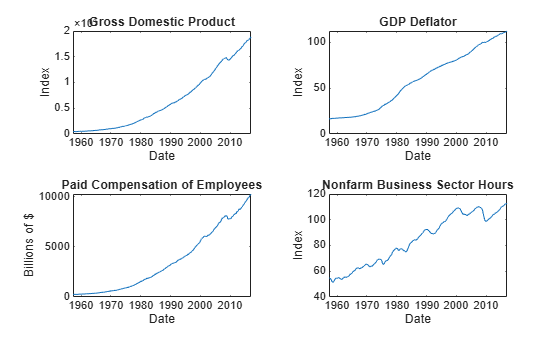```figure tiledlayout(2,2) nexttile plot(FRED.Time,FRED.FEDFUNDS) title("Federal Funds Rate") ylabel("Percent") xlabel("Date") nexttile plot(FRED.Time,FRED.PCEC) title("Consumption Expenditures") ylabel("Billions of \$") xlabel("Date") nexttile plot(FRED.Time,FRED.GPDI) title("Gross Private Domestic Investment") ylabel("Billions of \$") xlabel("Date")```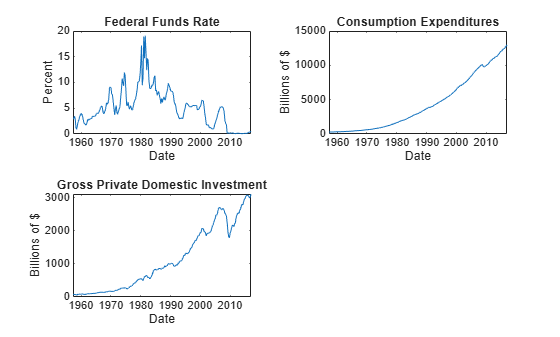Stabilize all series, except the federal funds rate, by applying the log transform. Scale the resulting series by 100 so that all series are on the same scale.

```FRED.GDP = 100*log(FRED.GDP); FRED.GDPDEF = 100*log(FRED.GDPDEF); FRED.COE = 100*log(FRED.COE); FRED.HOANBS = 100*log(FRED.HOANBS); FRED.PCEC = 100*log(FRED.PCEC); FRED.GPDI = 100*log(FRED.GPDI);```

Create a VEC(1) model using the shorthand syntax. Specify the variable names.

```Mdl = vecm(7,4,1); Mdl.SeriesNames = FRED.Properties.VariableNames```
```Mdl = vecm with properties: Description: "7-Dimensional Rank = 4 VEC(1) Model with Linear Time Trend" SeriesNames: "GDP" "GDPDEF" "COE" ... and 4 more NumSeries: 7 Rank: 4 P: 2 Constant: [7×1 vector of NaNs] Adjustment: [7×4 matrix of NaNs] Cointegration: [7×4 matrix of NaNs] Impact: [7×7 matrix of NaNs] CointegrationConstant: [4×1 vector of NaNs] CointegrationTrend: [4×1 vector of NaNs] ShortRun: {7×7 matrix of NaNs} at lag  Trend: [7×1 vector of NaNs] Beta: [7×0 matrix] Covariance: [7×7 matrix of NaNs] ```

`Mdl` is a `vecm` model object. All properties containing `NaN` values correspond to parameters to be estimated given data.

Estimate the model by supplying a matrix of data. Use default options.

`EstMdl = estimate(Mdl,FRED.Variables)`
```EstMdl = vecm with properties: Description: "7-Dimensional Rank = 4 VEC(1) Model" SeriesNames: "GDP" "GDPDEF" "COE" ... and 4 more NumSeries: 7 Rank: 4 P: 2 Constant: [14.1329 8.77841 -7.20359 ... and 4 more]' Adjustment: [7×4 matrix] Cointegration: [7×4 matrix] Impact: [7×7 matrix] CointegrationConstant: [-28.6082 109.555 -77.0912 ... and 1 more]' CointegrationTrend: [4×1 vector of zeros] ShortRun: {7×7 matrix} at lag  Trend: [7×1 vector of zeros] Beta: [7×0 matrix] Covariance: [7×7 matrix] ```

`EstMdl` is an estimated `vecm` model object. It is fully specified because all parameters have known values. By default, `estimate` imposes the constraints of the H1 Johansen VEC model form by removing the cointegrating trend and linear trend terms from the model. Parameter exclusion from estimation is equivalent to imposing equality constraints to zero.

Infer innovations from the estimated model, the residuals from the model fit. Supply the matrix of in-sample data.

`E = infer(EstMdl,FRED.Variables);`

`E` is a 238-by-7 matrix of inferred innovations. Columns correspond to the variable names in `EstMdl.SeriesNames`.

Alternatively, you can return residuals when you call `estimate` by supplying an output variable in the fourth position.

Plot the residuals on separate plots. Synchronize the residuals with the dates by removing the first `EstMdl.P` dates.

```idx = FRED.Time((EstMdl.P + 1):end); titles = "Residuals: " + EstMdl.SeriesNames; figure tiledlayout(2,2) for j = 1:4 nexttile plot(idx,E(:,j)) hold on yline(0,"r--") hold off title(titles(j)) end```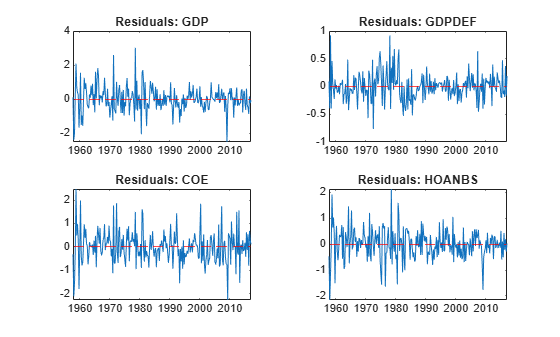```figure tiledlayout(2,2) for j = 5:7 nexttile plot(idx,E(:,j)) hold on yline(0,"r--") hold off title(titles(j)) end```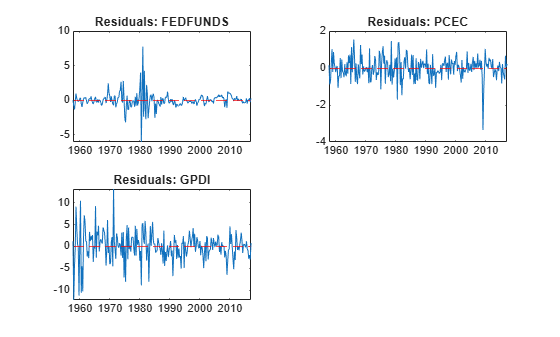The residuals corresponding to the federal funds rate exhibit heteroscedasticity.

Since R2022b

Consider a VEC model for the following seven macroeconomic series, and then fit the model to a timetable of response data. This example is based on Infer VEC Model Innovations From Matrix of Response Data.

Load the `Data_USEconVECModel` data set.

```load Data_USEconVECModel DTT = FRED; DTT.GDP = 100*log(DTT.GDP); DTT.GDPDEF = 100*log(DTT.GDPDEF); DTT.COE = 100*log(DTT.COE); DTT.HOANBS = 100*log(DTT.HOANBS); DTT.PCEC = 100*log(DTT.PCEC); DTT.GPDI = 100*log(DTT.GPDI);```

Prepare Timetable for Estimation

When you plan to supply a timetable directly to estimate, you must ensure it has all the following characteristics:

• All selected response variables are numeric and do not contain any missing values.

• The timestamps in the `Time` variable are regular, and they are ascending or descending.

Remove all missing values from the table.

```DTT = rmmissing(DTT); numobs = height(DTT)```
```numobs = 240 ```

`DTT` does not contain any missing values.

Determine whether the sampling timestamps have a regular frequency and are sorted.

`areTimestampsRegular = isregular(DTT,"quarters")`
```areTimestampsRegular = logical 0 ```
`areTimestampsSorted = issorted(DTT.Time)`
```areTimestampsSorted = logical 1 ```

`areTimestampsRegular = 0` indicates that the timestamps of DTT are irregular. `areTimestampsSorted = 1` indicates that the timestamps are sorted. Macroeconomic series in this example are timestamped at the end of the month. This quality induces an irregularly measured series.

Remedy the time irregularity by shifting all dates to the first day of the quarter.

```dt = DTT.Time; dt = dateshift(dt,"start","quarter"); DTT.Time = dt;```

`DTT` is regular with respect to time.

Create Model Template for Estimation

Create a VEC(1) model using the shorthand syntax. Specify the variable names.

```Mdl = vecm(7,4,1); Mdl.SeriesNames = DTT.Properties.VariableNames;```

`Mdl` is a `vecm` model object. All properties containing `NaN` values correspond to parameters to be estimated given data.

Fit Model to Data

Estimate the model by supplying the timetable of data `DTT`. By default, because the number of variables in `Mdl.SeriesNames` is the number of variables in `DTT`, `estimate` fits the model to all the variables in `DTT`.

`EstMdl = estimate(Mdl,DTT);`

`EstMdl` is an estimated `vecm` model object.

Compute Residuals

Infer innovations from the estimated model, the residuals from the model fit. Supply the timetable of in-sample data `DTT`. By default, because the number of variables in `Mdl.SeriesNames` is the number of variables in `DTT`, `infer` selects all the variables in `DTT`, from which to compute residuals.

```Tbl = infer(EstMdl,DTT); head(Tbl)```
``` Time GDP GDPDEF COE HOANBS FEDFUNDS PCEC GPDI GDP_Residuals GDPDEF_Residuals COE_Residuals HOANBS_Residuals FEDFUNDS_Residuals PCEC_Residuals GPDI_Residuals ___________ ______ ______ ______ ______ ________ ______ ______ _____________ ________________ _____________ ________________ __________________ ______________ ______________ 01-Jul-1957 617.44 281.55 558.01 399.59 3.47 566.71 437.32 0.12076 0.090979 -0.31114 -0.47341 -0.013177 0.14899 1.1764 01-Oct-1957 616.48 281.61 557.48 397.5 2.98 567.26 426.27 -2.4005 -0.39287 -2.1158 -2.1552 -0.86464 -0.89017 -12.289 01-Jan-1958 614.93 282.68 556.15 395.21 1.2 567.09 420.02 -2.0142 0.92195 -1.5874 -1.1852 -1.3247 -0.72797 -4.4964 01-Apr-1958 615.87 282.97 556.03 393.76 0.93 568.09 417.59 0.2131 -0.39586 -0.22658 -0.070487 -0.24993 0.17697 -0.31486 01-Jul-1958 618.76 283.57 558.99 394.95 1.76 569.81 427.67 2.0866 0.45876 2.4738 1.9098 0.98197 1.0195 9.119 01-Oct-1958 621.54 284.04 560.84 396.43 2.42 571.11 438.2 0.68671 0.053454 0.48556 0.63518 0.23659 -0.21548 4.2428 01-Jan-1959 623.66 284.31 563.55 398.35 2.8 573.62 442.12 0.39546 -0.066055 0.97292 1.0224 -0.054929 0.86153 0.68805 01-Apr-1959 626.19 284.46 565.91 400.24 3.39 575.54 449.31 0.24314 -0.22217 0.33889 0.4216 -0.20457 0.26963 -0.15985 ```
`size(Tbl)`
```ans = 1×2 238 14 ```

`Tbl` is a 238-by-14 timetable of in-sample data in `DTT` and estimated model residuals. Residual variables names are appended with `_Residuals`, for example, `GDP``_``Residuals`.

Alternatively, you can return residuals when you call `estimate` by supplying an output variable in the fourth position.

Since R2022b

Consider the model and data in Infer VEC Model Innovations From Matrix of Response Data.

Load the `Data_USEconVECModel` data set.

`load Data_USEconVECModel`

The `Data_Recessions` data set contains the beginning and ending serial dates of recessions. Load this data set. Convert the matrix of date serial numbers to a datetime array.

```load Data_Recessions dtrec = datetime(Recessions,ConvertFrom="datenum");```

Preprocess Data

Remove the exponential trend from the series, and then scale them by a factor of 100.

```DTT = FRED; DTT.GDP = 100*log(DTT.GDP); DTT.GDPDEF = 100*log(DTT.GDPDEF); DTT.COE = 100*log(DTT.COE); DTT.HOANBS = 100*log(DTT.HOANBS); DTT.PCEC = 100*log(DTT.PCEC); DTT.GPDI = 100*log(DTT.GPDI);```

Create a dummy variable that identifies periods in which the U.S. was in a recession or worse. Specifically, the variable should be `1` if `FRED.Time` occurs during a recession, and `0` otherwise. Include the variable with the `FRED` data.

```isin = @(x)(any(dtrec(:,1) <= x & x <= dtrec(:,2))); DTT.IsRecession = double(arrayfun(isin,DTT.Time));```

Prepare Timetable for Estimation

Remove all missing values from the table.

`DTT = rmmissing(DTT);`

To make the series regular, shift all dates to the first day of the quarter.

```dt = DTT.Time; dt = dateshift(dt,"start","quarter"); DTT.Time = dt;```

`DTT` is regular with respect to time.

Create Model Template for Estimation

Create a VEC(1) model using the shorthand syntax. Assume that the appropriate cointegration rank is 4. You do not have to specify the presence of a regression component when creating the model. Specify the variable names.

```Mdl = vecm(7,4,1); Mdl.SeriesNames = DTT.Properties.VariableNames(1:end-1);```

Fit Model to Data

Estimate the model using the entire sample. Specify the predictor identifying whether the observation was measured during a recession.

`EstMdl = estimate(Mdl,DTT,PredictorVariables="IsRecession");`

Compute Residuals

Infer innovations from the estimated model. Supply the predictor data. Return the loglikelihood objective function value.

```[Tbl,logL] = infer(EstMdl,DTT,PredictorVariables="IsRecession"); head(Tbl)```
``` Time GDP GDPDEF COE HOANBS FEDFUNDS PCEC GPDI IsRecession GDP_Residuals GDPDEF_Residuals COE_Residuals HOANBS_Residuals FEDFUNDS_Residuals PCEC_Residuals GPDI_Residuals ___________ ______ ______ ______ ______ ________ ______ ______ ___________ _____________ ________________ _____________ ________________ __________________ ______________ ______________ 01-Jul-1957 617.44 281.55 558.01 399.59 3.47 566.71 437.32 1 1.1766 0.1075 0.3528 0.15201 0.50983 0.75164 5.1297 01-Oct-1957 616.48 281.61 557.48 397.5 2.98 567.26 426.27 1 -1.2589 -0.375 -1.3979 -1.479 -0.29912 -0.23854 -8.014 01-Jan-1958 614.93 282.68 556.15 395.21 1.2 567.09 420.02 1 -1.2841 0.93338 -1.1283 -0.7527 -0.96303 -0.31126 -1.7628 01-Apr-1958 615.87 282.97 556.03 393.76 0.93 568.09 417.59 0 -0.30176 -0.40391 -0.55035 -0.37547 -0.50497 -0.11691 -2.2427 01-Jul-1958 618.76 283.57 558.99 394.95 1.76 569.81 427.67 0 1.872 0.4554 2.3388 1.7826 0.87564 0.89695 8.3152 01-Oct-1958 621.54 284.04 560.84 396.43 2.42 571.11 438.2 0 0.74477 0.054362 0.52207 0.66957 0.26535 -0.18234 4.4602 01-Jan-1959 623.66 284.31 563.55 398.35 2.8 573.62 442.12 0 0.52785 -0.063984 1.0562 1.1008 0.01065 0.93709 1.1838 01-Apr-1959 626.19 284.46 565.91 400.24 3.39 575.54 449.31 0 0.40825 -0.21958 0.44272 0.5194 -0.12278 0.36387 0.45836 ```
`logL`
```logL = -1.4656e+03 ```

`Tbl` is a 238-by-15 timetable of in-sample data in `DTT` and inferred innovations (variable names appended with `_Residuals`).

Plot the residuals on separate plots. Synchronize the residuals with the dates by removing the first `Mdl.P` dates.

```idx = endsWith(Tbl.Properties.VariableNames,"_Residuals"); resnames = Tbl.Properties.VariableNames(idx); titles = "Residuals: " + EstMdl.SeriesNames; figure tiledlayout(2,2) for j = 1:4 nexttile plot(Tbl.Time,Tbl{:,resnames(j)}) hold on yline(0,"r--") hold off title(titles(j)) end```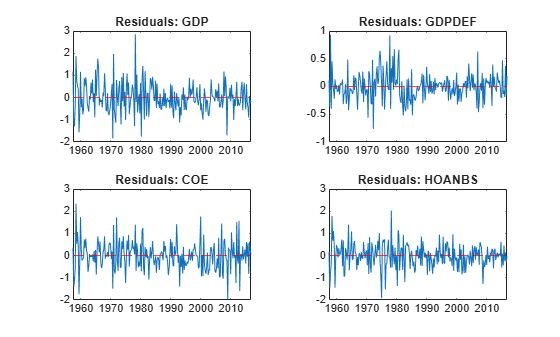```figure tiledlayout(2,2) for j = 5:7 nexttile plot(Tbl.Time,Tbl{:,resnames(j)}) hold on yline(0,"r--") hold off title(titles(j)) end```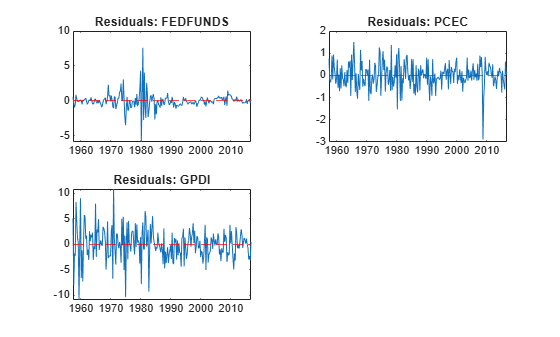The residuals corresponding to the federal funds rate exhibit heteroscedasticity.

## Input Arguments

collapse all

VEC model, specified as a `vecm` model object created by `vecm` or `estimate`. `Mdl` must be fully specified.

Response data, specified as a `numobs`-by-`numseries` numeric matrix or a `numobs`-by-`numseries`-by-`numpaths` numeric array.

`numobs` is the sample size. `numseries` is the number of response series (`Mdl.NumSeries`). `numpaths` is the number of response paths.

Rows correspond to observations, and the last row contains the latest observation. `Y` represents the continuation of the presample response series in `Y0`.

Columns must correspond to the response variable names in `Mdl.SeriesNames`.

Pages correspond to separate, independent `numseries`-dimensional paths. Among all pages, responses in a particular row occur at the same time.

Data Types: `double`

Since R2022b

Time series data containing observed response variables yt and, optionally, predictor variables xt for a model with a regression component, specified as a table or timetable with `numvars` variables and `numobs` rows.

Each selected response variable is a `numobs`-by-`numpaths` numeric matrix, and each selected predictor variable is a numeric vector. Each row is an observation, and measurements in each row occur simultaneously. You can optionally specify `numseries` response variables by using the `ResponseVariables` name-value argument, and you can specify `numpreds` predictor variables by using the `PredictorVariables` name-value argument.

Paths (columns) within a particular response variable are independent, but path `j` of all variables correspond, for `j` = 1,…,`numpaths`.

If `Tbl1` is a timetable, it must represent a sample with a regular datetime time step (see `isregular`), and the datetime vector `Tbl1.Time` must be ascending or descending.

If `Tbl1` is a table, the last row contains the latest observation.

### Name-Value Arguments

Specify optional pairs of arguments as `Name1=Value1,...,NameN=ValueN`, where `Name` is the argument name and `Value` is the corresponding value. Name-value arguments must appear after other arguments, but the order of the pairs does not matter.

Before R2021a, use commas to separate each name and value, and enclose `Name` in quotes.

Example: `infer(Mdl,Y,Y0=PS,X=Exo)` computes the residuals of the VEC(p – 1) model `Mdl` at the matrix of response data `Y`, and specifies the matrix of presample response data `PS` and the matrix of exogenous predictor data `Exo`.

Since R2022b

Variables to select from `Tbl1` to treat as response variables yt, specified as one of the following data types:

• String vector or cell vector of character vectors containing `numseries` variable names in `Tbl1.Properties.VariableNames`

• A length `numseries` vector of unique indices (integers) of variables to select from `Tbl1.Properties.VariableNames`

• A length `numvars` logical vector, where ```ResponseVariables(j) = true``` selects variable `j` from `Tbl1.Properties.VariableNames`, and `sum(ResponseVariables)` is `numseries`

The selected variables must be numeric vectors (single path) or matrices (columns represent multiple independent paths) of the same width, and cannot contain missing values (`NaN`).

If the number of variables in `Tbl1` matches `Mdl.NumSeries`, the default specifies all variables in `Tbl1`. If the number of variables in `Tbl1` exceeds `Mdl.NumSeries`, the default matches variables in `Tbl1` to names in `Mdl.SeriesNames`.

Example: `ResponseVariables=["GDP" "CPI"]`

Example: `ResponseVariables=[true false true false]` or `ResponseVariable=[1 3]` selects the first and third table variables as the response variables.

Data Types: `double` | `logical` | `char` | `cell` | `string`

Presample responses that provide initial values for the model `Mdl`, specified as a `numpreobs`-by-`numseries` numeric matrix or a `numpreobs`-by-`numseries`-by-`numprepaths` numeric array. Use `Y0` only when you supply a numeric array of response data `Y`.

`numpreobs` is the number of presample observations. `numprepaths` is the number of presample response paths.

Each row is a presample observation, and measurements in each row, among all pages, occur simultaneously. The last row contains the latest presample observation. `Y0` must have at least `Mdl.P` rows. If you supply more rows than necessary, `infer` uses the latest `Mdl.P` observations only.

Each column corresponds to the response series associated with the respective response series in `Y`.

Pages correspond to separate, independent paths.

• If `Y0` is a matrix, `infer` applies it to each path (page) in `Y`. Therefore, all paths in `Y` derive from common initial conditions.

• Otherwise, `infer` applies `Y0(:,:,j)` to `Y(:,:,j)`. `Y0` must have at least `numpaths` pages, and `infer` uses only the first `numpaths` pages.

By default, `infer` uses the first `Mdl.P` observations, for example, `Y(1:Mdl.P,:)`, as a presample. This action reduces the effective sample size.

Data Types: `double`

Since R2022b

Presample data that provides initial values for the model `Mdl`, specified as a table or timetable, the same type as `Tbl1`, with `numprevars` variables and `numpreobs` rows.

Each row is a presample observation, and measurements in each row, among all paths, occur simultaneously. `numpreobs` must be at least `Mdl.P`. If you supply more rows than necessary, `infer` uses the latest `Mdl.P` observations only.

Each variable is a `numpreobs`-by-`numprepaths` numeric matrix. Variables correspond to the response series associated with the respective response variable in `Tbl1`. To control presample variable selection, see the optional `PresampleResponseVariables` name-value argument.

For each variable, columns are separate, independent paths.

• If variables are vectors, `infer` applies them to each path in `Tbl1` to produce the corresponding residuals in `Tbl2`. Therefore, all response paths derive from common initial conditions.

• Otherwise, for each variable `ResponseK` and each path `j`, `infer` applies `Presample.ResponseK(:,j)` to produce `Tbl2.ResponseK(:,j)`. Variables must have at least `numpaths` columns, and `infer` uses only the first `numpaths` columns.

If `Presample` is a timetable, all the following conditions must be true:

• `Presample` must represent a sample with a regular datetime time step (see `isregular`).

• The inputs `Tbl1` and `Presample` must be consistent in time such that `Presample` immediately precedes `Tbl1` with respect to the sampling frequency and order.

• The datetime vector of sample timestamps `Presample.Time` must be ascending or descending.

If `Presample` is a table, the last row contains the latest presample observation.

By default, `infer` uses the first or earliest `Mdl.P` observations in `Tbl1` as a presample, and then it fits the model to the remaining `numobs – Mdl.P` observations. This action reduces the effective sample size.

Since R2022b

Variables to select from `Presample` to use for presample data, specified as one of the following data types:

• String vector or cell vector of character vectors containing `numseries` variable names in `Presample.Properties.VariableNames`

• A length `numseries` vector of unique indices (integers) of variables to select from `Presample.Properties.VariableNames`

• A length `numvars` logical vector, where ```PresampleResponseVariables(j) = true ``` selects variable `j` from `Presample.Properties.VariableNames`, and `sum(PresampleResponseVariables)` is `numseries`

The selected variables must be numeric vectors (single path) or matrices (columns represent multiple independent paths) of the same width, and cannot contain missing values (`NaN`).

`PresampleResponseNames` does not need to contain the same names as in `Tbl1`; `infer` uses the data in selected variable `PresampleResponseVariables(j)` as a presample for the response variable corresponding to `ResponseVariables(j)`.

The default specifies the same response variables as those selected from `Tbl1` (see `ResponseVariables`).

Example: ```PresampleResponseVariables=["GDP" "CPI"]```

Example: ```PresampleResponseVariables=[true false true false]``` or `PresampleResponseVariable=[1 3]` selects the first and third table variables for presample data.

Data Types: `double` | `logical` | `char` | `cell` | `string`

Predictor data xt for the regression component in the model, specified as a numeric matrix containing `numpreds` columns. Use `X` only when you supply a numeric array of response data `Y`.

`numpreds` is the number of predictor variables (`size(Mdl.Beta,2)`).

Each row corresponds to an observation, and measurements in each row occur simultaneously. The last row contains the latest observation. `X` must have at least as many observations as `Y`. If you supply more rows than necessary, `infer` uses only the latest observations. `infer` does not use the regression component in the presample period.

• If you specify a numeric array for a presample by using `Y0`, `X` must have at least `numobs` rows (see `Y`).

• Otherwise, `X` must have at least `numobs``Mdl.P` observations to account for the default presample removal from `Y`.

Each column is an individual predictor variable. All predictor variables are present in the regression component of each response equation.

`infer` applies `X` to each path (page) in `Y`; that is, `X` represents one path of observed predictors.

By default, `infer` excludes the regression component, regardless of its presence in `Mdl`.

Data Types: `double`

Since R2022b

Variables to select from `Tbl1` to treat as exogenous predictor variables xt, specified as one of the following data types:

• String vector or cell vector of character vectors containing `numpreds` variable names in `Tbl1.Properties.VariableNames`

• A length `numpreds` vector of unique indices (integers) of variables to select from `Tbl1.Properties.VariableNames`

• A length `numvars` logical vector, where `PredictorVariables(j) = true ` selects variable `j` from `Tbl1.Properties.VariableNames`, and `sum(PredictorVariables)` is `numpreds`

The selected variables must be numeric vectors and cannot contain missing values (`NaN`).

By default, `infer` excludes the regression component, regardless of its presence in `Mdl`.

Example: `PredictorVariables=["M1SL" "TB3MS" "UNRATE"]`

Example: `PredictorVariables=[true false true false]` or `PredictorVariable=[1 3]` selects the first and third table variables to supply the predictor data.

Data Types: `double` | `logical` | `char` | `cell` | `string`

Note

• `NaN` values in `Y`, `Y0`, and `X` indicate missing values. `infer` removes missing values from the data by list-wise deletion.

1. If `Y` is a 3-D array, then `infer` horizontally concatenates the pages of `Y` to form a `numobs`-by-```(numpaths*numseries + numpreds)``` matrix.

2. If a regression component is present, then `infer` horizontally concatenates `X` to `Y` to form a `numobs`-by-```numpaths*numseries + 1``` matrix. `infer` assumes that the last rows of each series occur at the same time.

3. `infer` removes any row that contains at least one `NaN` from the concatenated data.

4. `infer` applies steps 1 and 3 to the presample paths in `Y0`.

This process ensures that the inferred output innovations of each path are the same size and are based on the same observation times. In the case of missing observations, the results obtained from multiple paths of `Y` can differ from the results obtained from each path individually.

This type of data reduction reduces the effective sample size.

• `infer` issues an error when any table or timetable input contains missing values.

## Output Arguments

collapse all

Inferred multivariate innovations series, returned as either a numeric matrix, or as a numeric array that contains columns and pages corresponding to `Y`. `infer` returns `E` only when you supply a matrix of response data `Y`.

• If you specify `Y0`, then `E` has `numobs` rows (see `Y`).

• Otherwise, `E` has `numobs``Mdl.P` rows to account for the presample removal.

Since R2022b

Inferred multivariate innovations series and other variables, returned as a table or timetable, the same data type as `Tbl1`. `infer` returns `Tbl2` only when you supply the input `Tbl1`.

`Tbl2` contains the inferred innovation paths `E` from evaluating the model `Mdl` at the paths of selected response variables `Y`, and it contains all variables in `Tbl1`. `infer` names the innovation variable corresponding to variable `ResponseJ` in `Tbl1` `ResponseJ_Residuals`. For example, if one of the selected response variables for estimation in `Tbl1` is `GDP`, `Tbl2` contains a variable for the residuals in the response equation of `GDP` with the name `GDP_Residuals`.

If you specify presample response data, `Tbl2` and `Tbl1` have the same number of rows, and their rows correspond. Otherwise, because `infer` removes initial observations from `Tbl1` for the required presample by default, `Tbl2` has `numobs – Mdl.P` rows to account for that removal.

If `Tbl1` is a timetable, `Tbl1` and `Tbl2` have the same row order, either ascending or descending.

Loglikelihood objective function value, returned as a numeric scalar or a `numpaths`-element numeric vector. `logL(j)` corresponds to the response path in `Y(:,:,j)` or the path (column) `j` of the selected response variables of `Tbl1`.

## Algorithms

Suppose `Y`, `Y0`, and `X` are the response, presample response, and predictor data specified by the numeric data inputs in `Y`, `Y0`, and `X`, or the selected variables from the input tables or timetables `Tbl1` and `Presample`.

• `infer` infers innovations by evaluating the VEC model `Mdl`, specifically

`${\stackrel{^}{\epsilon }}_{t}=\stackrel{^}{\Phi }\left(L\right)\Delta {y}_{t}-\stackrel{^}{A}{\stackrel{^}{B}}^{\prime }{y}_{t-1}-\stackrel{^}{c}-\stackrel{^}{d}t-\stackrel{^}{\beta }{x}_{t}.$`

• `infer` uses this process to determine the time origin t0 of models that include linear time trends.

• If you do not specify `Y0`, then t0 = 0.

• Otherwise, `infer` sets t0 to `size(Y0,1)``Mdl.P`. Therefore, the times in the trend component are t = t0 + 1, t0 + 2,..., t0 + `numobs`, where `numobs` is the effective sample size (`size(Y,1)` after `infer` removes missing values). This convention is consistent with the default behavior of model estimation in which `estimate` removes the first `Mdl.P` responses, reducing the effective sample size. Although `infer` explicitly uses the first `Mdl.P` presample responses in `Y0` to initialize the model, the total number of observations in `Y0` and `Y` (excluding missing values) determines t0.

 Hamilton, James D. Time Series Analysis. Princeton, NJ: Princeton University Press, 1994.

 Johansen, S. Likelihood-Based Inference in Cointegrated Vector Autoregressive Models. Oxford: Oxford University Press, 1995.

 Juselius, K. The Cointegrated VAR Model. Oxford: Oxford University Press, 2006.

 Lütkepohl, H. New Introduction to Multiple Time Series Analysis. Berlin: Springer, 2005.